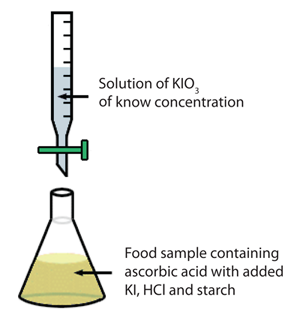# Iodometric Titration Tutorial

-Introduction

-Calculating moles of KIO3 required.

-Calculating concentration of KIO3 solution.

-Calculating mass of KIO3 to prepare solution.

-Conclusion

-Practice Question: Virtual Lab

Experiment 9 Iodometric Titration Online Tutorial >> Preparation of a KIO3 Standard Solution >> Introduction

 Next >

# Exp 9: Iodometric Titration Online Tutorial - Preparation of a KIO3 Standard Solution

## Introduction

A suitable method for the determination of vitamin C (C6H8O6) is a titration with potassium iodate (KIO3). Potassium iodate is used as a titrant and is added to an ascorbic acid solution that contains strong acid and potassium iodide (KI). Potassium iodate reacts with potassium iodide, liberating molecular iodine (I2):

    KIO3 + 5KI + 6H+ → 3I2 + 6K+ + 3H2O

As long as the solution contains ascorbic acid, the I2 produced in equation 1 is used up in a rapid reaction with ascorbic acid (equation 2), during which dehydroascorbic acid (C6H6O6) and iodide ion (I-) are formed:

    C6H8O6 + I2 → C6H6O6 + 2I- + 2H+

Potassium iodide must be added in excess to keep iodine dissolved. Once all the ascorbic acid has been consumed, any excess iodine will remain in solution. Since aqueous iodine solutions are brown in colour, iodine can act as its own indicator. However, it is quite difficult to detect endpoints using iodine colouration alone, and it is more usual to add starch, which forms an intensely blue coloured complex with iodine but not with the iodide ion.The experimental setup is shown schematically to the right.

To maintain 4 significant figures of accuracy in your measurements, the titration must use at least 10.00 mL of KIO3, and preferably 25 mL. As the amounts of vitamin C present in food samples can be quite small (<10 mg), a very dilute solution of KIO3 must be prepared. To ensure that the concentration of KIO3 is appropriately matched to the amount of ascorbic acid in your sample, you must first estimate that amount based on the sample you intend to investigate in your project. Let's assume that you estimated that about 10 mg of ascorbic acid is present in each sample you titrate. To determine the appropriate concentration of KIO3, we must follow the steps listed below:

Step 1

Calculate the number of moles of KIO3 required to completely react with the ascorbic acid present in a sample to be titrated.

Step 2

Calculate the concentration of KIO3 such that the number of moles calculated in Step 1 is contained in about 25 mL of KIO3 solution. That will ensure that about 25mL of KIO3 is used in each titration and so the 4 significant figures accuracy can be maintained.

Step 3

Calculate the mass of KIO3 required to prepare 250 mL of this solution.

The following pages contain tutors to assist you in the calculations required for the above steps. Please click next to continue to the tutors.

 Next >